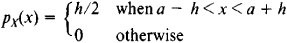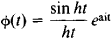# Uniform Distribution

(redirected from Boxcar distribution)

## uniform distribution

[′yü·nə‚fȯrm ‚di·strə′byü·shən]
(statistics)
The distribution of a random variable in which each value has the same probability of occurrence. Also known as rectangular distribution.
McGraw-Hill Dictionary of Scientific & Technical Terms, 6E, Copyright © 2003 by The McGraw-Hill Companies, Inc.
The following article is from The Great Soviet Encyclopedia (1979). It might be outdated or ideologically biased.

## Uniform Distribution

a special type of probability distribution of a random variable X that takes on values in the interval (a - h, a + h). A uniform distribution is characterized by the probability density functionThe mathematical expectation is EX = a, the variance is D X= h2/3, and the characteristic function isBy means of a linear transformation the interval (a - h, a + h) can be made to correspond to any given interval. Thus, the variable Y = (X - a + h)/2h is uniformly distributed over the interval (0, 1). Suppose the variables Y1, Y2,.…, Yn are uniformly distributed over the interval (0, 1). When their sum is normalized by the mathematical expectation n/2 and the variance n/12, the distribution law of the normalized sum rapidly approaches a normal distribution as n increases. In fact, the approximation is often sufficient for practical applications even when n = 3.